## Tangential Method Calculation

The tangential method is the simplest calculation among other directional survey calculations.The tangential method formulas are listed below;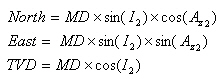Tangential Method Calculation formula

## What does the negative vertical section mean?

Vertical Section is the horizontal distance of wellbore that moves in the direction of the target per each station or in total.  For instance, in the figure below, vertical section is the distance from survey to survey point and it’s measured in the same direction of the vertical section direction.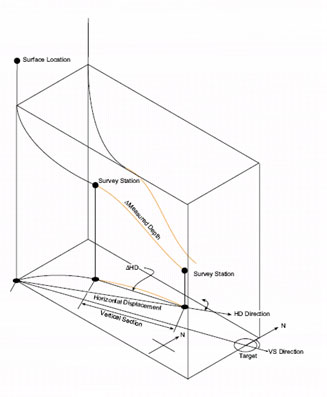The two factors that affect vertical section are as follows:

1. The Incremental horizontal displacement (? HD)

2. Vertical section direction (VSD) is the azimuth that is used to reference to the vertical section. Normally, VSD is the azimuth of the last target.

The simple mathematics as Average Angle Method calculation demonstrates the relationship of the VS as below:

VS = cos (VSD – Az avg) X ?HD

VS: Vertical Section

VSD: Vertical Section Direction

Az avg: Average Azimuth between 2 points (Az1 + Az2) ÷2

?HD: Delta Horizontal Displacement

In order to get the Positive Vertical Section or Zero Vertical Section, a well path must have difference of angle between VSD and Az avg, (VSD – Az avg), within a range of +90 to -90 degree. On the other hands, the negative Vertical Section can occur because the difference of angle between VSD and A zavg, (VSD – Az avg), is out of range of +90 to -90 degree AZI.

Radius of Curvature Method is the most famous method for directional survey calculation. You can calculate North, East and TVD between directional surveys based on the Radius of Curvature Method as per formula below: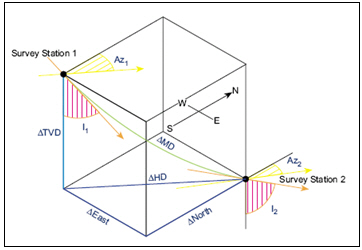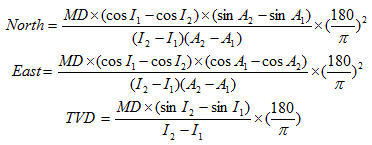Where;

MD = measured depth between surveys in ft

I1 = inclination (angle) at upper survey in degrees

I2 = inclination (angle) at lower in degrees

Az1= Azimuth direction at upper survey

Az2 = Azimuth direction at lower survey

Calculation example for Radius of Curvature Method

Survey 1

Depth = 7500 ft

Inclination = 45 degree (I1)

Azimuth = 130degree (A1)

Survey 2

Depth = 7595 ft

Inclination = 52 degree (I2)

Azimuth = 139 degree (A2)

MD = 7595 – 7500 = 95 ft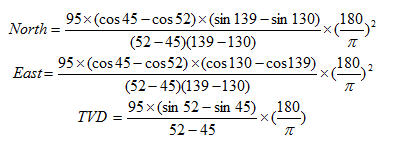As per the calculation above,

North = -49.97 ft

East = 50.66 ft

TVD = 62.91 ft

Please find the Excel sheet used for directional survey calculation with Radius of Curvature Method.

## Determine how many feet of free pipe without free pipe constant table

You may sometimes find the table for Free Point Constant for your stuck pipe calculation so you use the formula from the previous post to determine Free Point Constant and apply it into free pipe formula to determine where the depth of stuck pipe is.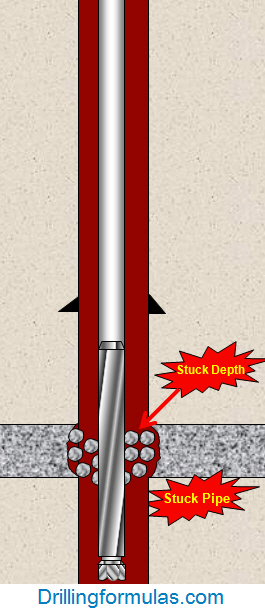Stuck Pipe Calculation – Stuck Depth

The steps of calculation are as follows:

1 ) Determine the free point constant (FPC) by this following formula:

FPC = As x 2500

where: As = pipe wall cross sectional area, sq in.

As = (OD2-ID2) x 0.7854

OD and ID are inch unit

2) Determine depth of stuck pipe by this following formula:

Depth of stuck pipe = (Pipe stretch in inch x free point constant (FPC)) ÷ Pull force in thousands of pounds

Please see the example below to demonstrate you how to apply those 2 formulas above into real drilling operation.

Example#2: Determine the free point constant (FPC) and the depth the pipe is stuck using the following information:

3-1/2” tubing # 9.5 lb/ft

3-1/2” tubing ID = 2.992 inch

20 inch of stretch with 25 Klb of pulling force

a) Determine free point constant (FPC):

FPC = (3.52 – 2.9922) x 0.7854 x 2500

FPC = 6475.5

b) Determine the depth of stuck pipe:

Feet of free pipe (ft) = (20 inch x 6475.5) ÷ 25

** 25 is the kilo-force to pull to 20 inch **

Feet of free pipe (ft) = 5,180 ft

Please find the Excel sheet used for calculating depth of stuck pipe.

## Determine how many feet of free pipe

Stuck pipe is one of serious situations that sometimes happen on the rig. People put a lot of effort to free stuck pipe; however, when they reach the point that they can not free the pipe anymore, they may need to know where the stuck point is in order to plan to cut or back off drill pipe. This post will demonstrate you how to determine free point constant and calculate where a stuck point is.

Firstly, we need to know the free point constant which can be found by this following table.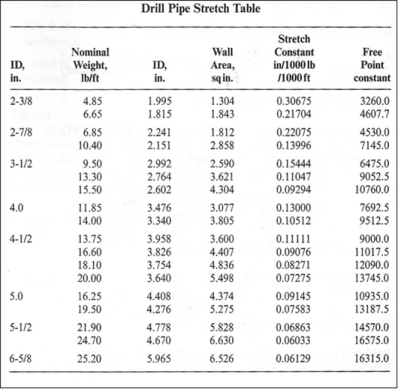Secondly, determine the depth where drill string gets stuck  by  the following formula.

Depth of stuck pipe in feet = (Pipe stretch in inch x free point constant (FPC)) ÷ Pull force in thousands of pounds

Example: Determine where the stuck pipe depth is by given information: 3-1/2 in. 9.5 ppf drill pipe, 25 inch of stretch with 30,000 lb of pull force

From drill pipe stretch table, the free point constant is 6475 for 3-1/2 in. drill pipe 9.5 ppf.

Feet of free pipe = (25 x 6475) ÷30

Feet of free pipe = 5396 ft

This mean there are 5396 ft of free drill pipe so when you know this figure, you can plan your wireline work for backing off drill pipe, colliding drill pipe, etc.

Please find the Excel sheet to calculate free pipe.

Ref books:

Lapeyrouse, N.J., 2002. Formulas and calculations for drilling, production and workover, Boston: Gulf Professional publishing.

Bourgoyne, A.J.T., Chenevert , M.E. & Millheim, K.K., 1986. SPE Textbook Series, Volume 2: Applied Drilling Engineering, Society of Petroleum Engineers.

Mitchell, R.F., Miska, S. & Aadny, B.S., 2011. Fundamentals of drilling engineering, Richardson, TX: Society of Petroleum Engineers.